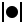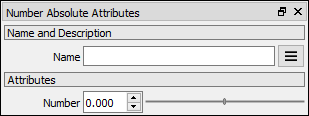# Number Absolute node

Left Toolbar >Math Nodes >Number Absolute

The Number Absolute node allows you to calculate the absolute value of a Number.

## Procedure

### To calculate the absolute value in the node graph:

1. On the left toolbar choose Math Nodes> Number Absolute2. Drag the Number Absolute icon into the Node Graph.3. Connect the Output: result.

## Inputs

number A NUMBER type of value.

## Outputs

Result The number value.

## Attributes PanelNumber Number value. This attribute is exposed as input by default.

Math Nodes

Main Page